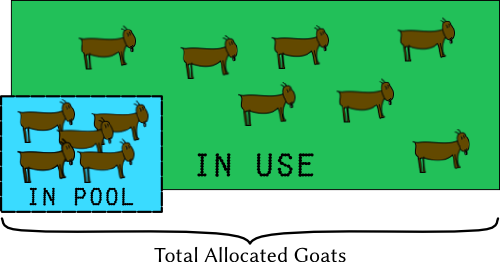08 February 2017### 写在前面

``````make([]int64, 0, len(ids))
``````

``````make([]int64, 0)
``````

``````a := make([]int64, 0)
fmt.Println(cap(a), len(a))

for i := 0; i < 3; i++ {
a = append(a, 1)
fmt.Println(cap(a), len(a))
}

0 0
1 1
2 2
4 3
``````

``````make([]int64, 0, len(ids))
``````

### 堆还是栈？

``````func F() {
temp := make([]int, 0, 20)
...
}
``````

``````func F() []int{
a := make([]int, 0, 20)
return a
}
``````

``````func F() {
a := make([]int, 0, 20)
b := make([]int, 0, 20000)

l := 20
c := make([]int, 0, l)
}
``````

`a``b`代码一样，就是申请的空间不一样大，但是它们两个的命运是截然相反的。`a`前面已经介绍过，会申请到栈上面，而`b`，由于申请的内存较大，编译器会把这种申请内存较大的变量转移到堆上面。即使是临时变量，申请过大也会在堆上面申请

`c`，对我们而言其含义和`a`是一致的，但是编译器对于这种不定长度的申请方式，也会在堆上面申请，即使申请的长度很短

``````go build -gcflags='-m' . 2>&1
``````

### 临时对象池

`func (p *Pool) Get() interface{}`

Get selects an arbitrary item from the Pool, removes it from the Pool, and returns it to the caller. Get may choose to ignore the pool and treat it as empty. Callers should not assume any relation between values passed to Put and the values returned by Get.

``````var idsPool = sync.Pool{
New: func() interface{} {
ids := make([]int64, 0, 20000)
return &ids
},
}

func NewIds() []int64 {
ids := idsPool.Get().(*[]int64)
*ids = (*ids)[:0]
idsPool.Put(ids)
return *ids
}
``````

``````var DEFAULT_SYNC_POOL *SyncPool

func NewPool() *SyncPool {
DEFAULT_SYNC_POOL = NewSyncPool(
5,
30000,
2,
)
return DEFAULT_SYNC_POOL
}

func Alloc(size int) []int64 {
return DEFAULT_SYNC_POOL.Alloc(size)
}

func Free(mem []int64) {
DEFAULT_SYNC_POOL.Free(mem)
}

// SyncPool is a sync.Pool base slab allocation memory pool
type SyncPool struct {
classes     []sync.Pool
classesSize []int
minSize     int
maxSize     int
}

func NewSyncPool(minSize, maxSize, factor int) *SyncPool {
n := 0
for chunkSize := minSize; chunkSize <= maxSize; chunkSize *= factor {
n++
}
pool := &SyncPool{
make([]sync.Pool, n),
make([]int, n),
minSize, maxSize,
}
n = 0
for chunkSize := minSize; chunkSize <= maxSize; chunkSize *= factor {
pool.classesSize[n] = chunkSize
pool.classes[n].New = func(size int) func() interface{} {
return func() interface{} {
buf := make([]int64, size)
return &buf
}
}(chunkSize)
n++
}
return pool
}

func (pool *SyncPool) Alloc(size int) []int64 {
if size <= pool.maxSize {
for i := 0; i < len(pool.classesSize); i++ {
if pool.classesSize[i] >= size {
mem := pool.classes[i].Get().(*[]int64)
// return (*mem)[:size]
return (*mem)[:0]
}
}
}
return make([]int64, 0, size)
}

func (pool *SyncPool) Free(mem []int64) {
if size := cap(mem); size <= pool.maxSize {
for i := 0; i < len(pool.classesSize); i++ {
if pool.classesSize[i] >= size {
pool.classes[i].Put(&mem)
return
}
}
}
}
``````

``````attrFilters := cache.Alloc(len(ids))
defer cache.Free(attrFilters)
``````

``````DEFAULT_SYNC_POOL = NewSyncPool(
5,
30000,
2,
)
``````
• 分配内存的时候，从池子里面找满足容量切最小的池子。比如申请长度是2的，就分配大小为5的那个池子。如果是11，就分配大小是20的那个池子里面的对象；
• 如果申请的`slice`很大，超过了上限30000，这种情况就不使用池子了，直接从内存申请；
• 当然这些参数可以根据自己实际情况调整；
• 和之前的做法有所区别，把对象重新放回池子是通过`Free`方法实现的。

### 结论

EOF

#### 欢迎加微信交流与吐槽